When I started my journey as a data scientist, I always put more focus on learning algorithms, that is the first mistake I made. Focusing too much on algorithms, while it's essential to know algorithms, is not that much important. For a machine learning project, the most important thing is data preparation. One of the vital parts of the data preparation is to treat missing values with the appropriate methods. Here we are trying to mimic the data generation process so, the understanding of the technique and how it works is paramount importance.

I learned this after spending endless hours on projects, but I'm glad that I learned this way because mistakes are a great way to learn. So this post is dedicated to data imputation methods that one can use for different types of variables.

Below we have a quick recap on the types of variables we have to deal with, in our machine learning project. Because the knowledge of these variables type will help us to understand the given method.Fig.1 Types of Variables

1) Complete Case Analysis (CCA):

This technique assumes that data points are missing at random, and it is suitable for both the categorical and numerical variables. In this technique, we drop the missing values while making sure that the distribution of that column remains the same. If the distribution changes after excluding the data, then this is a sign that we shouldn't be doing it.

1. This technique is easy to implement and quite fast.
2. This technique doesn't require any data manipulation.
3. One of the best things about this technique is that it maintains the distribution of the variables, thus, keeping the statistical properties intact to a certain extent.

1. If data contains too many missing instances, then this technique cannot be applied because that will lead to loss of information.
2. This analysis can create a biased dataset since dropping values may cause an increase in the percentage of other instances of categorical data.
Example:

Below is the example of a complete case analysis done on the Housing price data set. The first graph represents the histogram before dropping the nan-values while the second is the histogram after removing the nan-values while the 3rd is a combined graph to check how much distribution changes. Here we see that there is not much of a difference in the distribution. So, we can consider this variable for complete case analysis.

Similarly, we have two graphs one is before CCA, and the other is after CCA. We see that distribution looks the same. Hence, we can consider this variable for  CCA. Here is the jupyter notebook implementation of this technique.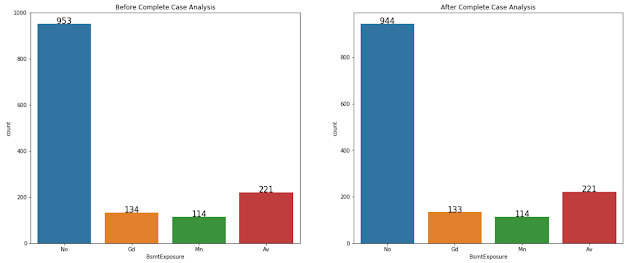Fig. 3 CCA on a Categorical Variable.

2) Mean, Median, and Mode Imputation (3M):

This technique uses a mean, median, or mode to replace the missing values in a dataset, Whether to use the mean, median, or mode depends on the data type of the variable. For example, for a continuous variable, we use mean, median, or mode while in a categorical or discrete variable, we use mode.
While performing mean, median, or mode imputation, we have to make sure that these values should be calculated on train data only and used for replacing the missing values in the train and test set. This way, we can avoid over-fitting.

1. This technique is lucid to apply also easy to implement during the model deployment process.
2. This technique doesn't require any data manipulation. It doesn't change the data frame.

1. This technique changes the distribution, which leads to a change in the statistical property like covariance, standard deviation, etc.
2. The higher the NA values higher the distortion, so this technique is not suitable when the percentage of missing values is high.
Example:

Here is the example of the "GaragYrBlt" variable of the house price dataset. In the following images, we can see the change in the distribution with different techniques. Here, we see that mean and median imputation have the same distribution, but that is not the case with mode imputation. The mode imputation fits almost perfectly with the original distribution.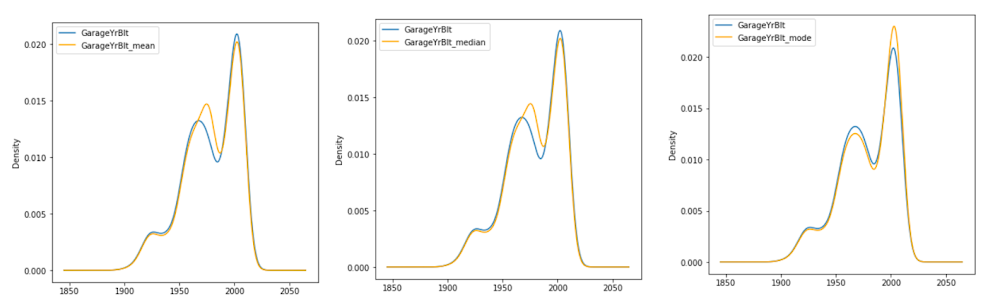Fig. 4 3M imputation

Above is the change in the statistical properties like standard deviation, median, and 75th percentile, etc. Here, is the jupyter notebook implementation of this technique.Fig. 5 Statistical Properties.

3) End Tail Imputation:

In this technique, we use end values or extreme values of the distribution to fill the missing values. This technique is only suitable for continuous data and not applicable to categorical data. While calculating end tail values, we have to make sure that it is computed only on the train set to avoid any data leakage else we may face the problem of over-fitting. In this technique, the end tails value depends on the kind of distribution followed by the variable.
In the case of normally distributed, we use Three standard deviations.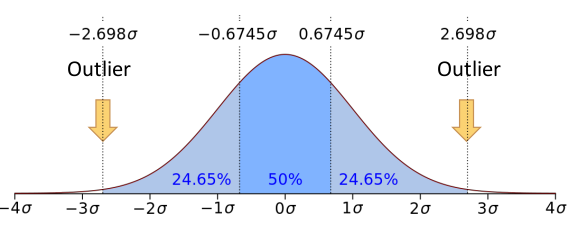Fig. 6 Normal Distribution.

Formula Used.Fig. 7 Formula.

In the case of a skewed normal distribution, we use the Interquartile range.Fig. 8 Skewed Distribution.

Formula Used.
1. This technique is easy to implement and integrates adeptly into production.
2. This technique doesn't introduce any outliers in the dataset.
3. This technique is quite robust for tree-based algorithms.

1. This technique distorts the original distribution hence changing the statistical relationship between the variables.
2. This technique may change the normality of the distribution. Thus, we avoid using it with linear models.
Example:

Below is the example of End tail imputation applied on the skewed dataset. Here we can see that how to whole distribution has changed by using lower or upper bound imputation. we see that the distribution changes from unimodal to bimodal.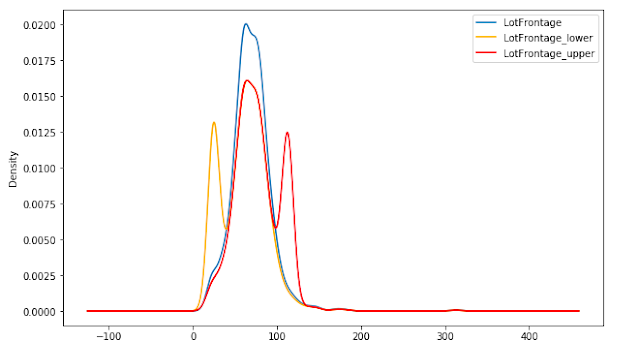Fig. 10   End tail Imputation.

Even when we look at the statistical properties of lower and upper imputation, we see that both are changing the statistical properties of the original variable., is the jupyter notebook implementation of this technique.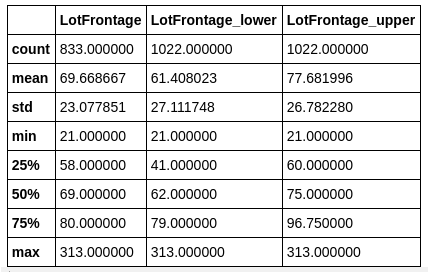Fig. 11 Statistical Properties

4) Missing Category Imputation:
It is a straightforward and effective technique when the missing data is high. In this technique, We replace missing values with the keyword "Missing"  or any word of choice we want. This technique applies to categorical data. The idea behind this technique is that we create an additional label of missing values. We can use this technique without splitting the data, but that is something one must avoid because it may create problems during model deployment.

1. It is easy to implement and easily deployable in production.
2. It captures the missingness of the data.
3. It is quite robust where missing data is quite high.

1. This technique fails when the number of missing values is small because then we are adding a rare label, that may affect the algorithm performance.
2. This technique increases the cardinality of the variable.
Example:
This the example of a categorical variable, here the "FirePlaceQu" variable has 46% of missing values so, it can be considered for the "Missing Tag Imputation". The first graph is the distribution before imputation while the second one is after the imputation. We see here that the "Missing" tag is the values that were missing and we filled them with the tag "Missing".Fig. 12 Missing Tag

Here, is the jupyter notebook implementation of this technique.

5) Random Sample Imputation:
In this technique, we use a random value to impute the missing values. The idea behind this technique is to maintain randomness in the data and not to introduce any biases. This technique is suitable for both numerical and categorical data. It assumes that data are missing at random.

1. It is easy to implement, and there is no data loss.
2. It is deployable in the machine learning pipeline during model deployment.
3. It is robust in the case of linear models.

1. If there are lots of missing values, this technique may impact the statistical relationship between the target and the variable.
2. It takes memory during model deployment since we have to store the training data to extract random values.
Example:

Here we have the "Lotfrontage" variable which is continuous in nature, and the 3 graphs representing the different scenarios. The first graph is plotted when no imputation was done on the data. The second graph is plotted after the random sample imputation. The third graph is a comparison that how distribution might change due to this imputation. Here we can observe that this imputation can keep the distribution intact to some extent.Fig. 13 Random sample imputation.

Here we have random sample imputation done on the categorical variable name "FireplaceQu". The first graph is the distribution of how the sales price changes with the different values of this category. The second graph is the distribution of sales price change after the imputation is done. We see that both the graph looks identical. Thus, we can use this technique for this variable.Fig. 14 Random Sample Imputation.

Here, is the jupyter notebook implementation of this technique.